Anúncio

# Signals and systems: part i solutions

23 de Mar de 2023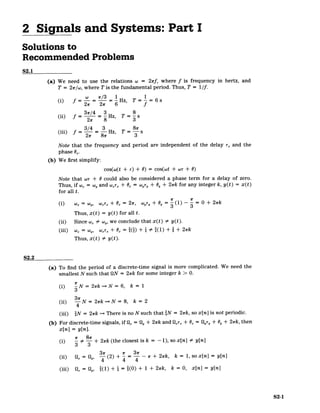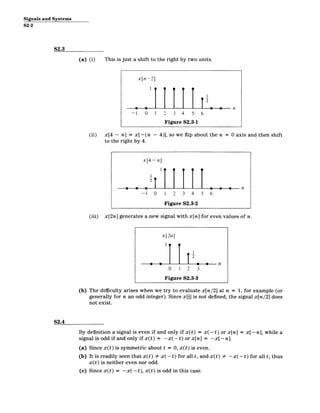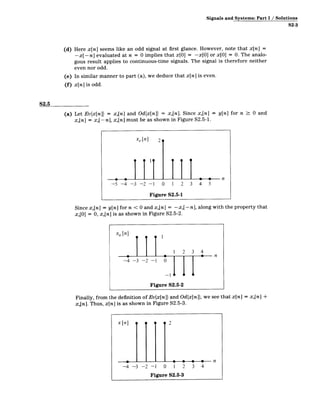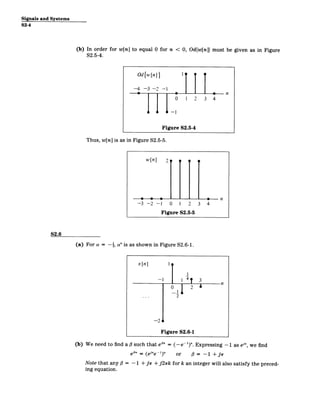Anúncio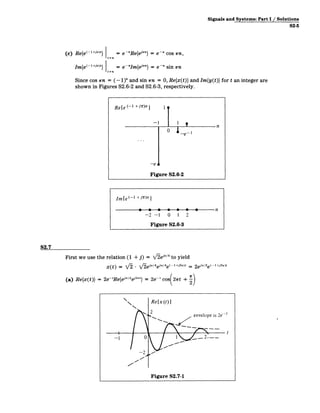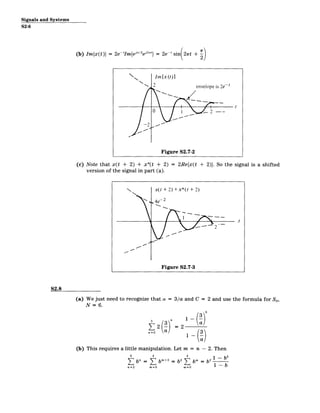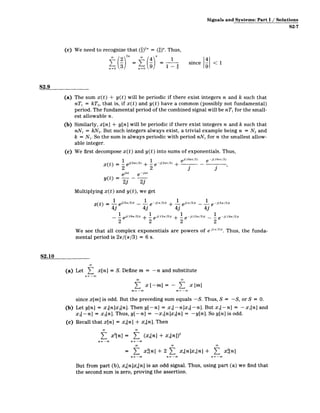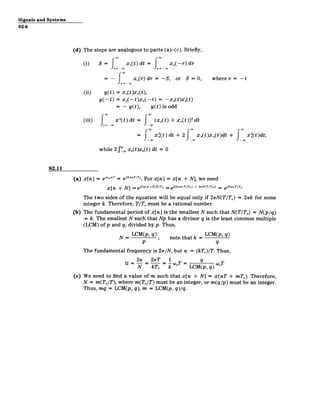Próximos SlideShares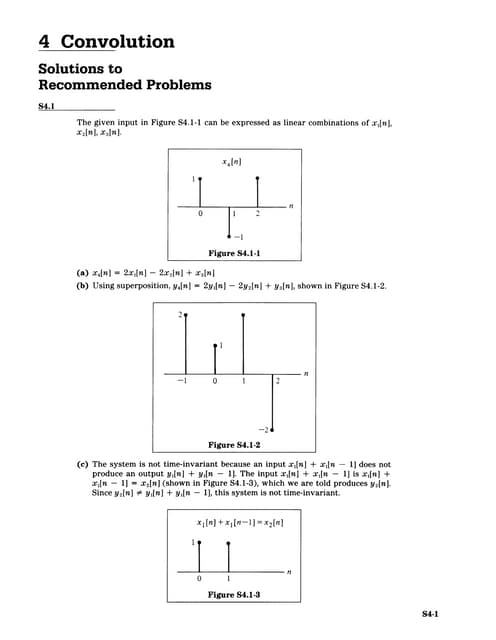convulution
Carregando em ... 3
1 de 9
Anúncio

### Signals and systems: part i solutions

1. 2 Signals and Systems: Part I Solutions to Recommended Problems S2.1 (a) We need to use the relations w = 21rf, where f is frequency in hertz, and T = 2w/w, where T is the fundamental period. Thus, T = 1/f. w /3 1 1 (i) f=-= =-Hz, T- 6s 2r 21 6 f 3r/4 3 8 (ii) f = =-Hz, T=-s 2w 8 3 3/4 3 8-x (iii) f = 2r = 87r Hz, T = 3 -s Note that the frequency and period are independent of the delay r, and the phase 0_. (b) We first simplify: cos(w(t + r) + 0) = cos(wt + wr + 0) Note that wT + 0 could also be considered a phase term for a delay of zero. Thus, if w, = w, and wX, + 0, = wor, + 6, + 2xk for any integer k, y(t) = x(t) for all t. (i) Wx = o ~ W, mr+Ox= 2 W, Wyr, +O ,= 3 1 - 3 =O0+ 2wk Thus, x(t) = y(t) for all t. (ii) Since wx # w,, we conclude that x(t) # y(t). (iii) COX= coy, omr, + 6X = ((i) + i #343(1) + a + 2,7k Thus, x(t) # y(t). S2.2 (a) To find the period of a discrete-time signal is more complicated. We need the smallest N such that UN = 21k for some integer k > 0. (i) N = 2wk =* N = 6, k = 1 3 (ii) N = 2rk =o N = 8, k = 2 4 (iii) 2N = 2wk => There is no N such that aN = 2wk, so x[n] is not periodic. (b) For discrete-time signals, if Ox = Q, + 2rk and Qxrx + Ox = Qr, + O,+ 2k, then x[n] = y[n]. (i) ' 81r + 2wk (the closest is k = -1), so x[n] # y[n] 3 3 (ii) Ox = I3, (2) + =r - r + 2k, k = 1, so x[n] = y[n] (iii) Ox= 1,,((1) + i = ((0) + 1 + 27rk, k = 0, x[n] = y[n] S2-1
2. Signals and Systems S2-2 S2.3 (a) (i) This is just a shift to the right by two units. x[n-2] -1 0 1 2 3 4 5 6 Figure S2.3-1 (ii) x[4 - n] = x[-(n - 4)], so we flip about the n = 0 axis and then shift to the right by 4. x[4-n] 111 0 0 2~l n -1 0 1 2 3 4 5 6 Figure S2.3-2 (iii) x[2n] generates a new signal with x[n] for even values of n. x [2n] 2 0 n 01 23 Figure S2.3-3 (b) The difficulty arises when we try to evaluate x[n/2] at n = 1, for example (or generally for n an odd integer). Since x[i] is not defined, the signal x[n/2] does not exist. S2.4 By definition a signal is even if and only if x(t) = x(-t) or x[n] = x[-n], while a signal is odd if and only if x(t) = -x(- t) or x[n] = -x[-n]. (a) Since x(t) is symmetric about t = 0, x(t) is even. (b) It is readily seen that x(t) # x(- t) for all t, and x(t) K -x(- t) for all t; thus x(t) is neither even nor odd. (c) Since x(t) = -x(- t), x(t) is odd in this case.
3. Signals and Systems: Part I / Solutions S2-3 (d) Here x[n] seems like an odd signal at first glance. However, note that x[n] = -x[-n] evaluated at n = 0 implies that x[O] = -x[O] or x[O] = 0. The analo­ gous result applies to continuous-time signals. The signal is therefore neither even nor odd. (e) In similar manner to part (a), we deduce that x[n] is even. (f) x[n] is odd. S2.5 (a) Let Ev{x[n]} = x[n] and Od{x[n]} = x[n]. Since xe[n] = y[n] for n >_0 and xe[n] = x[ -fn], x,[n] must be as shown in Figure S2.5-1. Xe[n] 2 4 1 4 n -5 -4 -3 -2 -1 0 1 2 3 4 5 Figure S2.5-1 Since x[n] = y[n] for n < 0 andx[n] = -x,[-n], along with the property that x0[O] = 0, x[n] is as shown in Figure S2.5-2. Xo [n] 1 2 3 4 0 n -4 -3 -2 -1 0 Figure S2.5-2 Finally, from the definition of Ev{x[n]} and Od{x[n]}, we see that x[n] = x,[n] + x[n]. Thus, x[n] is as shown in Figure S2.5-3.
4. Signals and Systems S2-4 (b) In order for w[n] S2.5-4. to equal 0 for n < 0, Od{w[n]} must be given as in Figure Odfw[n]} -4 - 3 --2 -- 0 14 1 2 3 4 n Figure S2.5-4 Thus, w[n] is as in Figure S2.5-5. w[n] 21 p -3 e -2 - -1 0 1 2 Figure S2.5-5 3 0 4 n S2.6 (a) For a = -ia"is as shown in Figure S2.6-1. x[n] 1 Figure S2.6-1 (b) We need to find a # such that e#' = (-e-')". Expressing -1 as ei", we find eon = (ej'e -)T or 0 = -1 + jr Note that any # = -1 + jfr + j27rk for k an integer will also satisfy the preced­ ing equation.
5. Signals and Systems: Part I / Solutions S2-5 (c) Re{e(-1 +')t) = e -Re{ej'"} = e -" cos rn, Im~e (- 1+j} n = e -"Im{e'"} = e~" sin in Since cos 7rn = (- 1)' and sin 7rn = 0, Re{x(t)) and Im{y(t)} for t an integer are shown in Figures S2.6-2 and S2.6-3, respectively. Refe (-1 + ir)n -1 1In n 0 -e­ -e Figure S2.6-2 Imfe (- +j7T)n I 0 0 0 0 0 -nf -2 -1 0 1 2 Figure S2.6-3 S2.7 First we use the relation (1 + j) = /Tej"!' to yield x(t) = / /ej'4eJ"/4e(-I+j2*)t = 2eir/2e(-1+j 2 )t (a) Re{x(t)} = 2e-'Re{ew"!ej'i'} = 2e-' cos( 2t + 2) Re{x (t)} envelope is 2e-r 2- -­ Figure S2.7-1
6. Signals and Systems S2-6 (b) Im{x(t)) = 2e-'Im{ejr/2 e 2 1r t } = 2e-' sin 27rt ImIx (t)} envelope is 2et 2 -­ Figure S2.7-2 (c) Note that x(t + 2) + x*(t + 2) = 2Re{x(t + 2)}. So the signal is a shifted version of the signal in part (a). x(t + 2) + x*(t + 2) , 4e 2 Figure S2.7-3 S2.8 (a) We just need to recognize that a = 3/a and C = 2 and use the formula for SN, N = 6. 6 =2 =32 -a) a /3 _ (3)a (b) This requires a little manipulation. Let m = n - 2. Then 6 4 4 1- 5 nb= nb= =0 bm ""2=b2(b=2 = ­ n=2 m=O M=0 1 -b
7. Signals and Systems: Part I / Solutions S2-7 (c) We need to recognize that (2)2n = (1)'. Thus, - - = o-4 since 1 S2.9 (a) The sum x(t) + y(t) will be periodic if there exist integers n and k such that nT1 = kT 2, that is, if x(t) and y(t) have a common (possibly not fundamental) period. The fundamental period of the combined signal will be nT1 for the small­ est allowable n. (b) Similarly, x[n] + y[n] will be periodic if there exist integers n and k such that nN = kN2. But such integers always exist, a trivial example being n = N2 and k = N1 . So the sum is always periodic with period nN, for n the smallest allow­ able integer. (c) We first decompose x(t) and y(t) into sums of exponentials. Thus, 1 1 ei(1 6 Tt/ 3 ) -j(16irt/3) x(t) = 1 ej( 2 1t/ 3 ) + -e j(21rt/3) + e e __A6r3 ____ 2 2 j Y( jrt -e-jrt 23 2j Multiplying x(t) and y(t), we get z(t) - e ( -+/3 - e-j5w/s) 7 )t( + / 3 )t -j 1/3 4j 4j 4j 4j 1 ej(1 9 r/ 3 )t+ 1 ej(13 r/3) t + 1 e j(13r/3)t - -j(19/3)t 2 2 2 2 We see that all complex exponentials are powers of e j(/3). Thus, the funda­ mental period is 2 7r/(7r/3) = 6 s. S2.10 (a) Let 7 x[n] = S. Define m = -n and substitute n = -oo Sx[-m] = - Z x[mI M= -OO M= -QO since x[m] is odd. But the preceding sum equals -S. Thus, S = -S, orS = 0. (b) Let y[n] = x 1[n]x2[n]. Then y[-n] = x1[-n]x 2[-n]. But x1[-nj = -xl[n] and x2[-n] = x 2[n]. Thus, y[-n] = -x1[n]x2[n] = -y[n]. So y[n] is odd. (c) Recall that x[n] = x[fn] + x[n]. Then E x2[n] = E (xe[nl + x.[n])2 = x[n] + 2 E Xe[flx]Xfl + >7 x2[n] n= -co n= o n= -0 But from part (b), x,[nxo[n] is an odd signal. Thus, using part (a) we find that the second sum is zero, proving the assertion.
8. Signals and Systems S2-8 (d) The steps are analogous to parts (a)-(c). Briefly, (i) S =f x0(t) dt = x,(-r)dr =0­ x 0(r) dr = -S, or S = 0, where r = -t (ii) (iii) y(t) = Xo(t)xe(lt), y(-t) = xo(-t)x(-t) - y(t), x 2(t) dt = f = -xo(t)xo(t) y(t) is odd (X(t) + x0(t)) 2 dt = x(t ) dt + 2 Xe(t)xo(t)dt + x 2(t)dt, while 2r x(t)xo(t) dt = 0 S2.11 (a) (b) x[n] = ewonT - ei2 nTTo. For x[n] = x[n + N], we need x[n + N] = ej2 x(n +N)T/To eji[ 2 n(T/To) + 2rN(T/To)] = ej2nT/To The two sides of the equation will be equal only if 27rN(T/TO) = 27rk for some integer k. Therefore, TITO must be a rational number. The fundamental period of x[n] is the smallest N such that N(T/TO) = N(p/q) = k. The smallest N such that Np has a divisor q is the least common multiple (LCM) of p and q, divided by p. Thus, N LCM(p, q); p note that k = LCM(p, q) q The fundamental frequency is 2ir/N, but n = (kT0)/T. Thus, (c) Q= 2 = = WT = q T N kT,, k LCM(p, q) 0 We need to find a value of m such that x[n + N] = x(nT + mT). Therefore, N = m(T./T), where m(T./T) must be an integer, or m(q/p) must be an integer. Thus, mq = LCM(p, q), m = LCM(p, q)/q.
9. MIT OpenCourseWare http://ocw.mit.edu Resource: Signals and Systems Professor Alan V. Oppenheim The following may not correspond to a particular course on MIT OpenCourseWare, but has been provided by the author as an individual learning resource. For information about citing these materials or our Terms of Use, visit: http://ocw.mit.edu/terms.
Anúncio